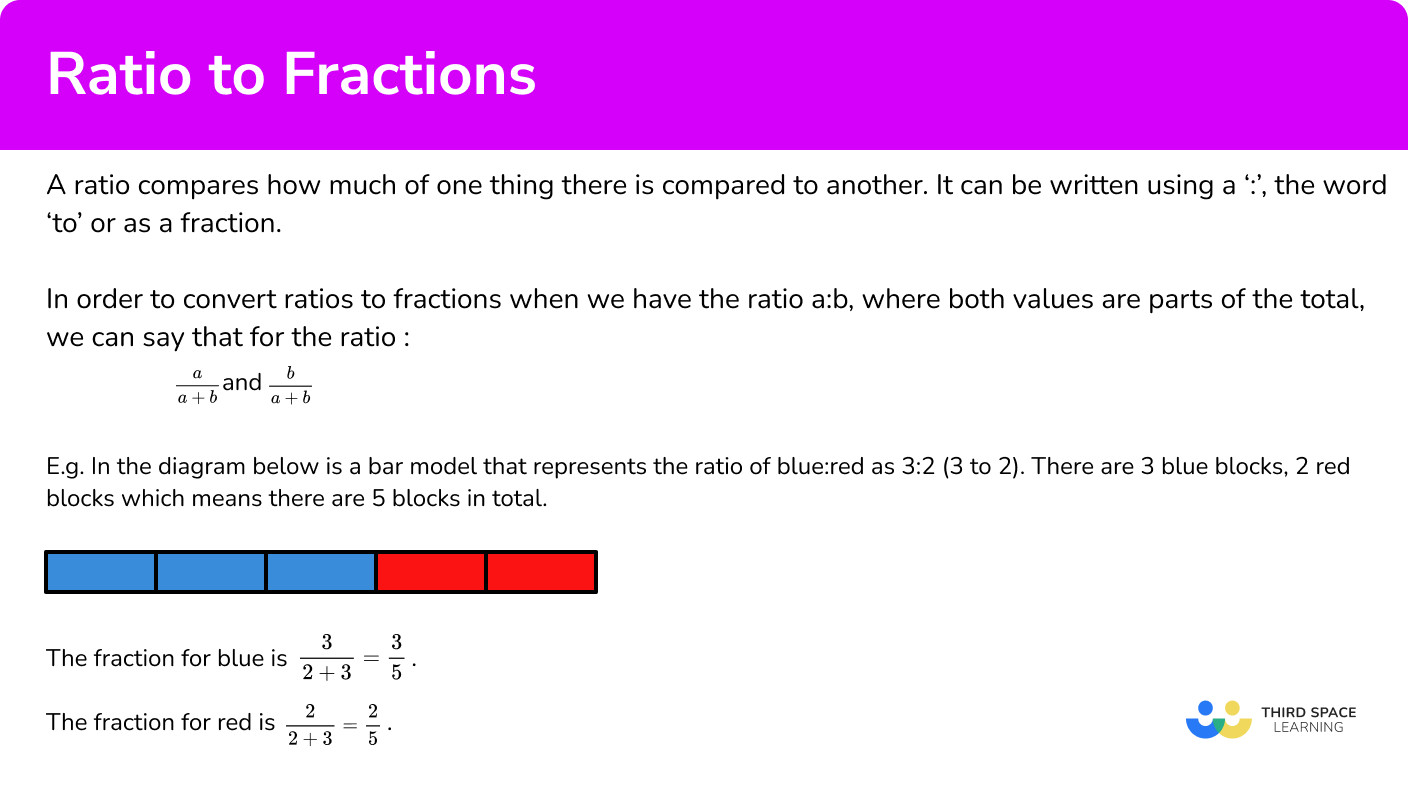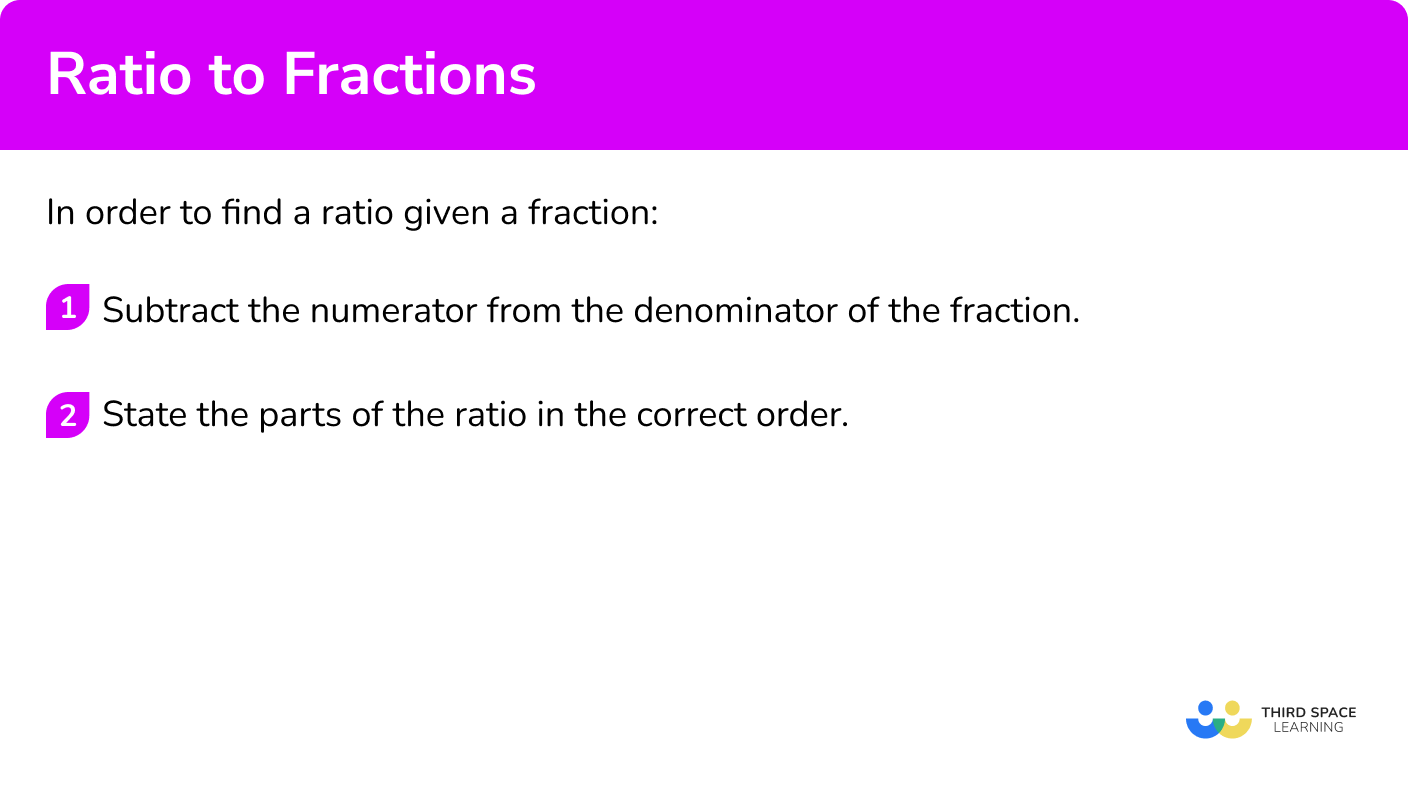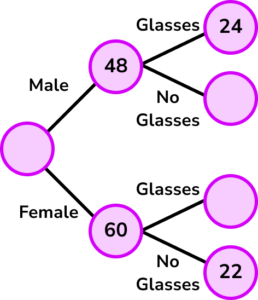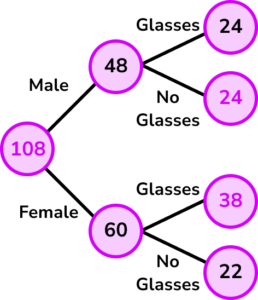# Ratio To Fraction

Here we will learn about ratios to fractions, including using ratios to find fractions and using fractions to find ratios.

There are also ratio to fractions worksheets based on Edexcel, AQA and OCR exam questions, along with further guidance on where to go next if you’re still stuck.

## What is a ratio to a fraction?

A ratio to fraction is a way of writing a ratio as a fraction. A ratio compares how much of one thing there is compared to another. It can be written using a ‘:’, the word ‘to’ or as a fraction.

In order to convert ratios to fractions when we have the ratio a:b, where both values are parts of the total, we can say that for the ratio  \frac{a}{a+b} and \frac{b}{a+b}.

E.g.

In the diagram below is a bar model that represents the ratio of blue:red as 3:2 (3 to 2). There are 3 blue blocks, 2 red blocks which means there are 5 blocks in total.

The fraction for blue is \frac{3}{2+3}=\frac{3}{5}.

The fraction for red is \frac{2}{2+3}=\frac{2}{5}.

Here the total number of shares in the ratio is equal to a+b (the denominator of each fraction) and the numerator is the part of the ratio we are interested in.

Note: if it is possible to simplify the fraction (or the ratio) then simplify it but remember to keep using whole numbers (integers).

Step-by-step guide: How to work out ratios (coming soon)

### What is a ratio to a fraction?## How to find a fraction given a ratio

In order to find a fraction given a ratio:

1. Add the parts of the ratio for the denominator.
2. State the required part of the ratio as the numerator.

### Explain how to find a fraction given a ratio## Related lessons on ratio

Ratio to fraction is part of our series of lessons to support revision on ratio. You may find it helpful to start with the main ratio lesson for a summary of what to expect, or use the step by step guides below for further detail on individual topics. Other lessons in this series include:

## Ratio to fraction examples

### Example 1: standard question

Ann and Bob share a box of cookies in the ratio of 3:4. What fraction of the cookies does Bob receive?

1. Add the parts of the ratio for the denominator.

3+4=7

2State the required part of the ratio as the numerator.

Solution: \frac{4}{7}

### Example 2: picture

The diagram below shows part of the repeating pattern of red and yellow beads on a bracelet.

What fraction of the beads in the bracelet are red?

Add the parts of the ratio for the denominator.

State the required part of the ratio as the numerator.

### Example 3: three part ratio

A tin of paint requires white, yellow and blue paint in the ratio 5:3:2.

What fraction of the tin is blue paint?

Add the parts of the ratio for the denominator.

State the required part of the ratio as the numerator.

### Example 4: bar modelling

A group of friends want to watch a film at the cinema. Below is a bar model to represent the friends’ choices. The friends who want to watch a romantic comedy are in red, those who want to watch a science fiction film are represented by yellow, and the others want to watch the latest action blockbuster.

What fraction of the group of friends do not want to watch a science fiction film? Write your answer in its simplest form.

Add the parts of the ratio for the denominator.

State the required part of the ratio as the numerator.

## How to find a ratio given a fraction

In order to find a ratio given a fraction:

1. Subtract the numerator from the denominator of the fraction.
2. State the parts of the ratio in the correct order.

### Explain how to find a ratio given a fraction## Fraction to ratio examples

### Example 5: standard question

\frac{3}{4} of a school of fish are male. The rest are female. Write the ratio of females to males in the school.

Subtract the numerator from the denominator of the fraction.

State the parts of the ratio in the correct order.

### Example 6: worded problem

Pepper the cat spends roughly \frac{3}{5} of each day sleeping. State the ratio of hours awake to hours asleep to hours asleep for Pepper.

Subtract the numerator from the denominator of the fraction.

State the parts of the ratio in the correct order.

### Example 7: three part ratio

A dental practice sells 3 different packages of dental care plans: Basic, Premium, and Family. \frac{1}{4} of their members have the Basic plan, whilst \frac{1}{5} have a Premium plan. State the ratio of Basic : Premium : Family.

Subtract the numerator from the denominator of the fraction.

State the parts of the ratio in the correct order.

### Common misconceptions

• Ratios and fractions confusion

For example, the ratio 2:3 is expressed as the fraction \frac{2}{3} and not \frac{2}{5}. This is a misunderstanding of the sum of the parts of the ratio. Be careful with what the question is asking as the denominator may be the part, or the whole amount.

• Mixing units

Make sure that all the units in the ratio are the same. For example, in example 6, all the units in the ratio were in millilitres. We did not mix ml and l in the ratio.

• Incorrect value for the numerator

The numerator is incorrectly stated from the ratio. For example, the number of mugs to glasses in a kitchen is written as the ratio 4:3 respectively. Write the fraction of mugs in the kitchen. The correct answer is \frac{4}{7}. The “mugs” value is the first number in the ratio, m:g=4:3 as we use the same order as the written sentence.

• Ratio written in the wrong order

The parts of the ratio are written in the wrong order. For example the number of dogs to cats is given as the ratio 12:13 but the solution is written as 13:12.

### Practice ratio to fraction questions

1. The ratio of tyrannosaurus rex to velociraptor fossils is 3:8. What fraction of the fossils are tyrannosaurus rex? Give your answer as a fraction in its simplest form.

\frac{3}{8}\frac{8}{11}\frac{3}{11}\frac{8}{3}Total number of fossils = 3+8=11

3 out of 11 are T-Rex fossils so \frac{3}{11}

2. A tailor sells silk and polyester ties in a ratio of 8:7. Calculate the fraction of ties that are silk.

\frac{8}{7}\frac{7}{8}\frac{7}{15}\frac{8}{15}8+7=15

8 out of 15 ties are silk so \frac{8}{15}.

3. The HEX colour \#428715 used for websites is made from the ratio of red to green to blue as 66:135:21 respectively. What fraction of the colour is green? Simplify your answer.\frac{135}{222}\frac{45}{74}\frac{135}{21}\frac{135}{66}66+135+21=222

135 out of 222 are green so \frac{135}{222} = \frac{45}{74}.

4. There are 52 cards in a deck. \frac{12}{52} cards are picture cards. State the ratio of picture cards to non-picture cards in its simplest form.

3:103:1310:313:352-12=40

picture:not picture

12:40=3:10 simplified

5. A football team won \frac{3}{5} of their matches in the league. They did not draw any matches. Write the ratio of wins : losses.

3:23:53:0:22:35-3=2

Win : Lose = 3:2

6. The number of beetles (B), slugs (S) and worms (W) eaten by a hedgehog was recorded over one night. \frac{1}{6} of the insects eaten were beetles, and \frac{2}{9} were slugs. Give the ratio of insects eaten by the hedgehog B:S:W in the simplest form.

3:4:111:2:61:2:311:3:4\frac{1}{6}+\frac{2}{9}=\frac{3}{18}+\frac{4}{18}=\frac{7}{18}

1-\frac{7}{18}=\frac{11}{18}

3:4:11

### Ratio to fraction GCSE questions

1. A market stall sells apples, pears and bananas only. The ratio of apples to pears is given as mixed numbers 5:2:1. Write down the fraction of the apples sold.

(2 marks)

5+2+1=8

(1)

\frac{5}{8}

(1)

2. (a) The school orchestra is playing in a concert. 30\% of ticket sales were sold to members of the public (P), \ \frac{3}{5} sales were to family and friends of the musicians (F), and the rest were sold to staff at the school (S).
Write the number of ticket sales as a ratio in its simplest form P:F:S

(b) If 440 tickets were sold to the public and staff, how many tickets were sold to members of the public only?

(4 marks)

(a)

\frac{3}{5}=60\%, \ 60\%+30\%=90\%

(1)

3:6:1

(1)

(b)

440 \div (3+1)=110

(1)

110 \times 3=330

(1)

3. (a) Complete the frequency tree using the information provided.(b) Write the ratio of people that wear glasses to the total number of people in the simplest form.

(5 marks)

(a)(3)

(b)

38+24=62 wear glasses

(1)

62:108 = 31:54

(1)

## Learning checklist

You have now learned how to:

• Understand that 2 or more quantities can be expressed as a ratio or a fraction

## Still stuck?

Prepare your KS4 students for maths GCSEs success with Third Space Learning. Weekly online one to one GCSE maths revision lessons delivered by expert maths tutors.

Find out more about our GCSE maths tuition programme.